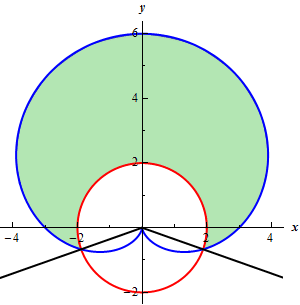Paul's Online Notes
Home / Calculus II / Parametric Equations and Polar Coordinates / Area with Polar Coordinates
Show Mobile Notice Show All Notes Hide All Notes
Mobile Notice
You appear to be on a device with a "narrow" screen width (i.e. you are probably on a mobile phone). Due to the nature of the mathematics on this site it is best views in landscape mode. If your device is not in landscape mode many of the equations will run off the side of your device (should be able to scroll to see them) and some of the menu items will be cut off due to the narrow screen width.

### Section 9.8 : Area with Polar Coordinates

3. Find the area that is inside $$r = 3 + 3\sin \theta$$ and outside $$r = 2$$.

Show All Steps Hide All Steps

Start Solution

First, here is a quick sketch of the graph of the region we are interested in.Show Step 2

Now, we’ll need to determine the values of $$\theta$$ where the graphs intersect (indicated by the black lines on the graph in the previous step).

There are easy enough to find. Because they are where the graphs intersect we know they must have the same value of $$r$$. So,

\begin{align*}3 + 3\sin \theta & = 2\\ \sin \theta & = - \frac{1}{3}\hspace{0.5in} \Rightarrow \hspace{0.5in}\theta = {\sin ^{ - 1}}\left( { - \frac{1}{3}} \right) = - 0.3398\end{align*}

This is the angle in the fourth quadrant where the graphs intersect.

From a quick sketch of a unit circle we can quickly get the angle in the third quadrant where the two graphs intersect.

$\theta = \pi + 0.3398 = 3.4814$

The ranges of $$\theta$$ for this problem is then $$- 0.3398 \le \theta \le 3.4814$$.

Show Step 3

From the graph we can see that $$r = 3 + 3\sin \theta$$ is the “outer” graph for this region and $$r = 2$$ is the “inner” graph.

The area is then,

\begin{align*}A & = \int_{{ - 0.3398}}^{{3.4814}}{{\frac{1}{2}\left[ {{{\left( {3 + 3\sin \theta } \right)}^2} - {{\left( 2 \right)}^2}} \right]\,d\theta }}\\ & = \frac{1}{2}\int_{{ - 0.3398}}^{{3.4814}}{{5 + 18\sin \theta + 9{{\sin }^2}\left( \theta \right)\,d\theta }}\\ & = \frac{1}{2}\int_{{ - 0.3398}}^{{3.4814}}{{5 + 18\sin \theta + \frac{9}{2}\left( {1 - \cos \left( {2\theta } \right)} \right)\,d\theta }}\\ & = \frac{1}{2}\int_{{ - 0.3398}}^{{3.4814}}{{\frac{{19}}{2} + 18\sin \theta - \frac{9}{2}\cos \left( {2\theta } \right)\,d\theta }}\\ & = \left. {\frac{1}{2}\left( {\frac{{19}}{2}\theta - 18\cos \left( \theta \right) - \frac{9}{4}\sin \left( {2\theta } \right)} \right)} \right|_{ - 0.3398}^{3.4814} = \require{bbox} \bbox[2pt,border:1px solid black]{{33.7074}}\end{align*}

Make sure you can do the trig manipulations required to do these integrals. Most of the integrals in this section will involve this kind of manipulation. If you don’t recall how to do them go back and take a look at the Integrals Involving Trig Functions section.